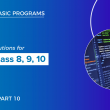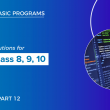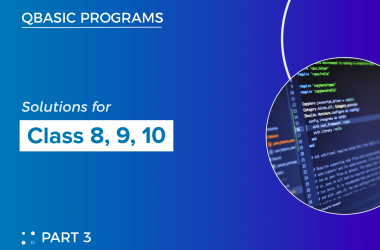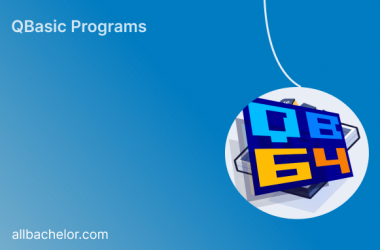# Qbasic programs solutions(part 11) for class 8, class 9 and class 10

Here, I have listed some of the qbasic programs. We also have mobile app where you can view all the qbasic programs. For better experience I highy recommend you to use our mobile app

201  Write a program in qbasic to ask number and check whether the given no is prime or not

``````CLS
INPUT "ENTER ANY NUMBER"; N
C = 0
FOR I = 1 TO N
IF N MOD I = 0 THEN C = C + 1
NEXT I
IF C = 2 THEN
PRINT N; "IS PRIME NUMBER"
ELSE
PRINT N; "IS NOT PRIME NUMBER"
END IF
END ``````

202  Write a program in qbasic to generate the series 1   2   4   8   16… upto 10th term

``````CLS
a = 1
FOR i = 1 TO 10
PRINT a
a = a * 2
NEXT i
END ``````

203  Write a program in qbasic to convert decimal number to binary number

``````CLS
INPUT "ENTER DECIMAL NUMBER"; D
WHILE D <> 0
R = D MOD 2
S\$ = STR\$(R) + S\$
D = D \ 2
WEND
PRINT "BINARY EQUIVALENT VALUE="; S\$
END ``````

204  Write a program in qbasic to generate the series 50   46   41   35…………7th terms

``````CLS
A = 50
B = 4
FOR I = 1 TO 7
PRINT A
A = A - B
B = B + 1
NEXT I
END ``````

205  Write a program in qbasic to generate the series 1   4   7   10…………12th terms

``````CLS
A = 1
FOR I = 1 TO 12
PRINT A;
A = A + 3
NEXT I
END ``````

206   Write a program in qbasic to print all palindrome numbers from 1 to 200

``````CLS
N = 1
FOR I = 1 TO 200
A = N
S = 0
WHILE A  <> 0
R = A MOD 10
S = S * 10 + R
A = A \ 10
WEND
IF N = S THEN PRINT N,
N = N + 1
NEXT I
END``````

207  Write a program in qbasic to print all natural numbers from 1 to 100

``````CLS
FOR I = 1 TO 100
PRINT I,
NEXT I
END ``````

208  Write a program in qbasic to ask any string and print only consonants

``````CLS
INPUT "ENTER ANY STRING"; S\$
FOR I = 1 TO LEN(S\$)
B\$ = MID\$(S\$, I, 1)
C\$ = UCASE\$(B\$)
IF C\$  <> "A" AND C\$  <> "E" AND C\$ <> "I" AND C\$ <> "O"
AND C\$ <> "U" AND C\$  <> " " AND C\$  <> "." THEN
PRINT B\$
END IF
NEXT I
END ``````

209   Write a program in qbasic to print first 15 prime numbers

``````CLS
INPUT "ENTER ANY STRING"; S\$
FOR I = 1 TO LEN(S\$)
B\$ = MID\$(S\$, I, 1)
C\$ = UCASE\$(B\$)
IF C\$  <> "A" AND C\$  <> "E" AND C\$ <> "I" AND C\$ <> "O"
AND C\$ <> "U" AND C\$  <> " " AND C\$  <> "." THEN
PRINT B\$
END IF
NEXT I
END ``````

210  Write a program in qbasic to generate the following series ½    2/3    ¾………15th term

``````CLS
A = 1
B = 2
FOR I = 1 TO 15
PRINT A; "/"; B
A = A + 1
B = B + 1
NEXT I
END ``````

211  Write a program in qbasic to print first 20 palindrome numbers

``````CLS
N = 1
CNT = 1
TOP:
A = N
S = 0
WHILE A <> 0
R = A MOD 10
S = S * 10 + R
A = A \ 10
WEND
IF N = S THEN
PRINT N,
CNT = CNT + 1
END IF
N = N + 1
IF CNT  <= 20 THEN GOTO TOP
END ``````

212  Write a program in qbasic to ask any number and print the factors

``````CLS
INPUT "ENTER ANY NUMBER"; N
PRINT "FACTORS OF"; N; "=";
FOR I = 1 TO N
IF N MOD I = 0 THEN PRINT I;
NEXT I
END ``````

213  Write a program in qbasic to ask any word and count total no of vowels and consonants

``````CLS
INPUT "ENTER ANY WORD"; S\$
VC = 0
CC = 0
FOR I = 1 TO LEN(S\$)
B\$ = MID\$(S\$, I, 1)
C\$ = UCASE\$(B\$)
IF C\$ = "A" OR C\$ = "E" OR C\$ = "I" OR C\$ = "O" OR C\$ = "U" THEN
VC = VC + 1
ELSE
CC = CC + 1
END IF
NEXT I
PRINT "TOTAL NO. OF VOWELS= "; VC
PRINT "TOTAL NO. OF CONSONANTS="; CC
END ``````

214  Write a program in qbasic to ask three angles of a triangle and determine whether a triangle is right angled triangle or not

``````CLS
INPUT “ENTER THREE ANGLES OF A TRIANGLE”; A,B,C
IF A = 90 OR B = 90 OR C = 90 THEN
PRINT “IT IS A RIGHT ANGLED TRIANGLE”
ELSE
PRINT “IT IS NOT A RIGHT ANGLED TRIANGLE”
END IF
END
﻿``````

215  Write a program in qbasic to print 3   6   12   24………15th terms

``````CLS
A = 3
FOR I = 1 TO 15
PRINT A
A = A * 2
NEXT I
END ``````

216  Write a program in qbasic to generate the series 1   5   25   125….10th terms

``````CLS
A = 1
FOR I = 1 TO 10
PRINT A,
A = A * 5
NEXT I
END ``````

217  Write a program to enter any three numbers and display the middle number.

``````CLS
INPUT “ENTER ANY THREE NUMBERS”; A, B, C
IF A  > B AND A  < C OR A  <  B AND A > C THEN
PRINT A; “IS MIDDLE NUMBER”
ELSEIF B  > A AND B < C OR B <  A AND B > C THEN
PRINT B; “IS MIDDLE NUMBER”
ELSE
PRINT C; “IS MIDDLE NUMBER”
END IF
END ``````

218  Write a program in qbasic TO ENTER ANY DIGIT AND PRINT CUBE OF EVEN DIGITS

``````CLS
INPUT "ENTER ANY NUMBER"; N
S = 0
WHILE N  <> 0
R = N MOD 10
IF R MOD 2 = 0 THEN S = S + R ^ 3
N = N \ 10
WEND
PRINT "SUM OF CUBE OF EVEN DIGITS"; S
END``````

219  Write a program to enter any 10 numbers and display the greatest one.

``````CLS
INPUT “ENTER ANY THREE NUMBERS”; A, B, C
IF A > B AND A > C THEN
PRINT A; “IS GREATEST”
ELSEIF B > A AND B > C THEN
PRINT B; “IS GREATEST”
ELSE
PRINT C; “IS GREATEST”
END IF
END
﻿``````

220  Write a program in qbasic TO ENTER ANY DIGIT AND PRINT SQUARE OF ODD

``````CLS
INPUT "ENTER ANY NUMBER"; N
PRINT "SQUARE OF ODD DIGITS ARE ";
WHILE N  <> 0
R = N MOD 10
IF R MOD 2 = 1 THEN PRINT R ^ 2;
N = N \ 10
WEND
END
﻿``````

1.Vikas kumar Verma says:

सर बीएससी फर्स्ट ईयर की नोट्स कंप्यूटर प्रोग्रामिंग इन बेसिक पास्कल मिल सकता है

1.Bishal Khadka says:

Thank you for your interest. I will be constantly updating this site.

##### Previous Post## Qbasic programs solutions(part 10) for class 8, class 9 and class 10

##### Next Post## Qbasic programs solutions(part 12) for class 8, class 9 and class 10

##### Related Posts## Qbasic programs solutions(part 3) for class 8, class 9 and class 10

Here, I have listed some of the qbasic programs. We also have mobile app where you can view…## QBASIC in Modern Times

Introduction QBASIC, a programming language born in the early 1980s, has retained its nostalgic charm and enduring appeal.…## A Journey Through QBASIC and Other Programming Languages: A Comprehensive Comparison

Introduction Programming languages form the backbone of modern software development, enabling developers to create diverse applications and solve…